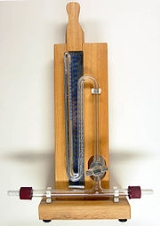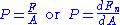xPressureOverview

Pressure is the force per unit area
Area
Area is a quantity that expresses the extent of a two-dimensional surface or shape in the plane. Area can be understood as the amount of material with a given thickness that would be necessary to fashion a model of the shape, or the amount of paint necessary to cover the surface with a single coat...

applied in a direction perpendicular
Perpendicular
In geometry, two lines or planes are considered perpendicular to each other if they form congruent adjacent angles . The term may be used as a noun or adjective...

to the surface of an object. Gauge pressure
Pressure measurement
Many techniques have been developed for the measurement of pressure and vacuum. Instruments used to measure pressure are called pressure gauges or vacuum gauges....

is the pressure relative to the local atmospheric or ambient pressure.
Pressure is the effect of a force applied to a surface. Pressure is the amount of force acting per unit area. The symbol of pressure is P.
Mathematically:where:
P is the pressure,
F is the normal force
Normal force
In mechanics, the normal force F_n\ is the component, perpendicular to the surface of contact, of the contact force exerted on an object by, for example, the surface of a floor or wall, preventing the object from penetrating the surface.The normal force is one of the components of the ground...

,
A is the area of the surface area on contact

Pressure is a scalar
Scalar (physics)
In physics, a scalar is a simple physical quantity that is not changed by coordinate system rotations or translations , or by Lorentz transformations or space-time translations . This is in contrast to a vector...

quantity.